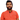# Learn Pain LessHomeOur TeamContact
Python
What Does // Mean in Python: Understanding this Common OperatorPawneshwer Gupta
March 07, 2023
2 min

01
Understanding the Basics of the // Operator
02
Use Cases of the // Operator in Python
03
ConclusionAt first glance, the `//` operator in Python may seem confusing. However, it is an important operator that you will frequently encounter in Python programming. In this article, we will dive deep into what does // mean in python and how it can be used.

## .css-1qh5hbx{box-sizing:border-box;margin:0;min-width:0;display:block;color:var(--theme-ui-colors-heading,#2d3748);font-weight:bold;-webkit-text-decoration:none;text-decoration:none;margin-bottom:1rem;font-size:1.5rem;position:relative;}Understanding the Basics of the // Operator

what is // in python: The `//` operator is known as the integer division operator in Python. It is used to perform division between two numbers and return the result as an integer. In Python, there are two division operators, namely the `/` operator and the `//` operator. The `/` operator returns a float value, whereas the `//` operator returns an integer value.

#### Let’s take an example to understand this better. Consider the following code snippet:

```.css-1baulvz{display:inline-block;}a = 10b = 3
print(a/b) # Output: 3.3333333333333335print(a//b) # Output: 3```

In the above code, we have two variables `a` and `b`. When we perform division between `a` and `b` using the `/` operator, we get a float value as the output. However, when we perform division between `a` and `b` using the `//` operator, we get an integer value as the output.

## Use Cases of the // Operator in Python

The `//` operator is commonly used in situations where we need to perform division and get the quotient as an integer value. One such example is when we want to calculate the number of times a smaller value can fit into a larger value. Let’s take an example to understand this:

```total = 20batch_size = 3
num_batches = total // batch_sizeprint(num_batches) # Output: 6```

In the above code, we have two variables `total` and `batch_size`. We want to calculate the number of batches we can create given the total number of items and batch size. We use the `//` operator to perform integer division and get the result as an integer value.

Another use case of the `//` operator is when we want to perform floor division. Floor division is the division of two numbers rounded down to the nearest integer. Let’s take an example to understand this:

```a = -10b = 3
print(a/b) # Output: -3.3333333333333335print(a//b) # Output: -4```

In the above code, we have two variables `a` and `b`. When we perform division between `a` and `b` using the `/` operator, we get a float value as the output. However, when we perform division between `a` and `b` using the `//` operator, we get the result rounded down to the nearest integer, which is `-4`.

## Conclusion

In conclusion, the `//` operator in Python is an important operator that is used to perform integer division between two numbers. It is commonly used in situations where we need to get the quotient as an integer value. It is also used when we want to perform floor division. Understanding the `//` operator is crucial for anyone who wants to learn what is // in python, What Does // Mean in Python and Python programming.

We'll send you the best of our blog just once a month. We promise.

Operator

## Software Developer

Pawneshwer Gupta works as a software engineer who is enthusiastic in creating efficient and innovative software solutions.

Python
Flutter
Laravel
NodeJS

## Related PostsUnderstanding Python deque (Double-Ended Queue)
August 23, 2023
2 min